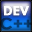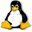Home Latest News Archive Tutorials C++ 2 3 4 Win32 Java OpenGL GLUT OpenGL ES 2 3 4 DirectX VB.NET HTML Photoshop 3dsmax Forums Projects Modelling Links About Us Search Recent Tutorials C / C++ Win32 OpenGL OpenGL ES GLUT## Recursion

Tutorials > C++ > Recursion

### What is Recursion?

Recursion occurs when a function calls itself.

eg.void func() { func(); }

This is a very bad function as the function will loop forever, constantly calling itself. Eventually, stack space will run out and the program will crash. There therefore needs to be a stopping condition. This condition should be chosen so that the program will always reach this condition no matter what parameters are passed onto the program. You do not want to have your program crashing every 2nd time you run it.

### Why is Recursion useful?

You may have a problem that requires a typical operation to act on a piece of data a number of times. Most recursive functions can be converted into an iterative function (loops), but recursion is usually a more simple approach. You shall see how recursion can be used below.

Contents of main.cpp :

```#include <iostream>
#include <stdlib.h>

using namespace std;
```

Our first recursive function calculates the factorial of a number. A factorial of a number N is calculated as follows :

N! = N * (N-1) * (N-2) * ... * 1

```int factorial(int num)
{
```

Below we provide our stopping condition. We carry on multiplying the current number by the previous value - 1 and eventually the value passed onto the function will be 1. We therefore return 1 as this is what the previously calculated value should be multiplied by in the last step.

```	if (num == 1)
return 1;
```

The line below is what makes this function a recursive one. We take the value passed onto the function and multiply it by the same value - 1. To make this clearer, we can look at how 3! is calculated.

 num Parameter Return Value 3 3 * factorial(2) 2 2 * factorial(1) 1 1

We can see that factorial(1) returns 1 and factorial(2) returns 2 * factorial(1).
factorial(2) therefore returns 2 * 1 which is 2.
Our original calculation is 3 * factorial(2) which from the above calculation returns 3 * 2 which is 6.
Our final value returned is therefore 6.

```	return num * factorial(num - 1);
}
```

Another example of recursion is given below where the power of a number is calculated eg. 2 to the power of 3 is 8. The function takes as its first parameter, the base number and the second parameter is the exponent.

```int power(int base, int index)
{
```

Our stopping condition is similar to the factorial function above.

```	if (!index)
return 1;
```

Our recursive call is shown below. The base is multiplied by the power of the same base to the same exponent - 1 eg.

2^3 = 2^1 * 2^2

```	return base * power(base, index - 1);
}
```

The functions are tested below.

5! = 120

2^8 = 256

```int main()
{
cout << factorial(5) << endl;

cout << power(2, 8) << endl;

system("pause");

return 0;
}```

Well done. You should now have an understanding of what recursion is and how it works. Remember not to let your function recurse too much as each time you call the function, new variables are pushed onto the stack. If you run out of space on the stack, your program will crash. Recursion can often make complex problems easily solvable.

Please let me know of any comments you may have : Contact Me

 Source Files :< Tutorial 33 - C++ Strings Tutorial 35 - Random Numbers >